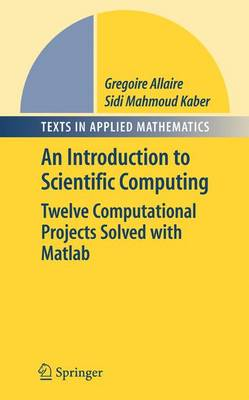•# Numerical Linear Algebra - Texts in Applied Mathematics 55 (Hardback)

(author), (translator), (author)
£74.99
Hardback 271 Pages / Published: 05/12/2007
• We can order this from the publisher

Usually dispatched within 4-6 weeks

This book distinguishes itself from the many other textbooks on the topic of linear algebra by including mathematical and computational chapters along with examples and exercises with Matlab. In recent years, the use of computers in many areas of engineering and science has made it essential for students to get training in numerical methods and computer programming. Here, the authors use both Matlab and SciLab software as well as covering core standard material. It is intended for libraries; scientists and researchers; pharmaceutical industry.

Publisher: Springer-Verlag New York Inc.
ISBN: 9780387341590
Number of pages: 271
Weight: 1280 g
Dimensions: 235 x 155 x 17 mm

MEDIA REVIEWS

From the reviews:

"Allaire (Ecole Polytechnique, France) and Kaber (Universite Pierre et Marie Curie, France) taught a numerical linear algebra course to third-year undergraduates; this work, initially published in French, is the culmination of that course. ... What sets this book apart from others on the subject is its experimental approach in all exercises. For many exercises, the book provides complete solutions including MATLAB scripts ... . Summing Up: Recommended. Upper-division undergraduates, researchers/faculty, and professionals/practitioners." (J. T. Zerger, CHOICE, Vol. 45 (10), June, 2008)

"The book is concerned with two main aspects from the field of matrix computations: solving linear systems of equations and, respectively, computing eigenvalues and eigenvectors of matrices. It is intended for advanced undergraduate students ... ." (Constantin Popa, Zentralblatt MATH, Vol. 1135 (13), 2008)

"The textbook Numerical linear algebra is the English translation of the French edition with the same title ... . The book is divided into eleven chapters. At the end of each chapter some relevant test problems are given. ... The material presented in the book is very well-organized. ... This book is an excellent tool for teachers and students." (Zahari Zlatev, Mathematical Reviews, Issue 2008 m)

"The present book covers material adequate for students at the end of their undergraduate or the beginning of their graduate years. ... The presentation is clear and mathematically precise, and solutions to the numerous exercises ... are provided. Overall, this is a clear and useful introduction into numeral linear algebra." (H. Muthsam, Monatshefte fur Mathematik, Vol. 158 (3), November, 2009)

"This book ... was developed for use in a course on numerical linear algebra for third-year undergraduates. ... The methods are described briefly but clearly. ... The exercises are one of the main strengths of the book. ... I can recommend the book as a textbook for undergraduates." (James E. Gentle, SIAM Review, Vol. 52 (1), 2010)